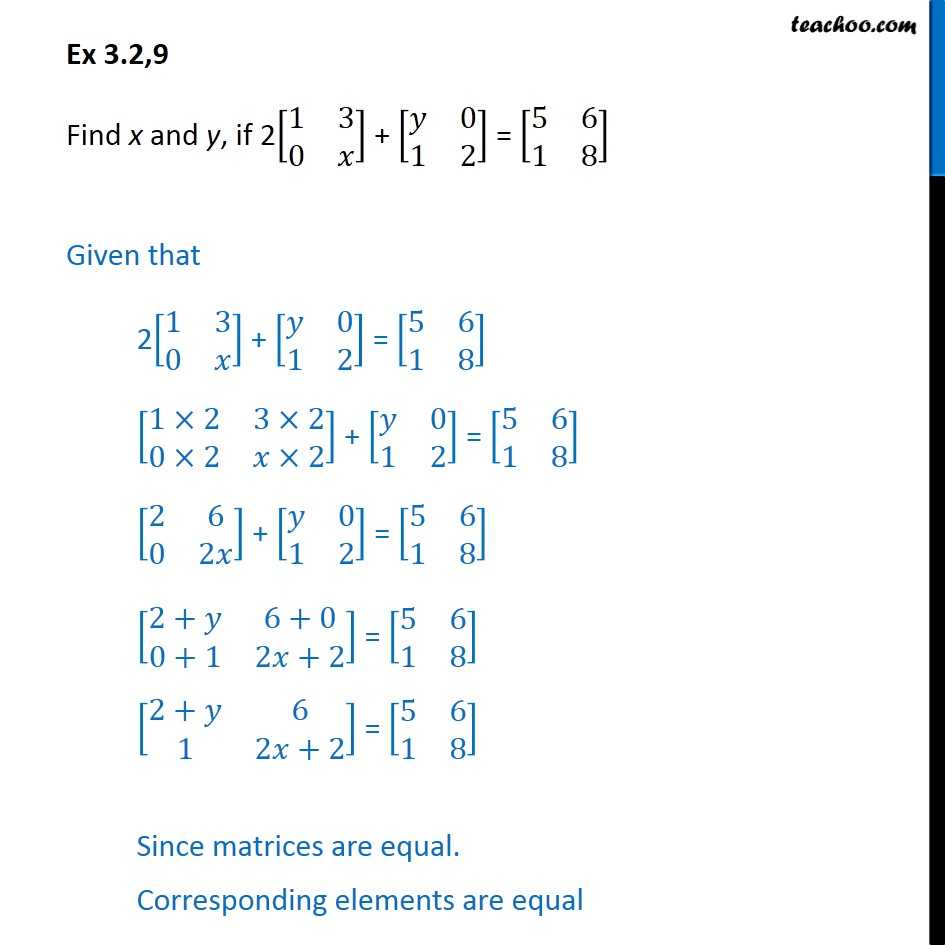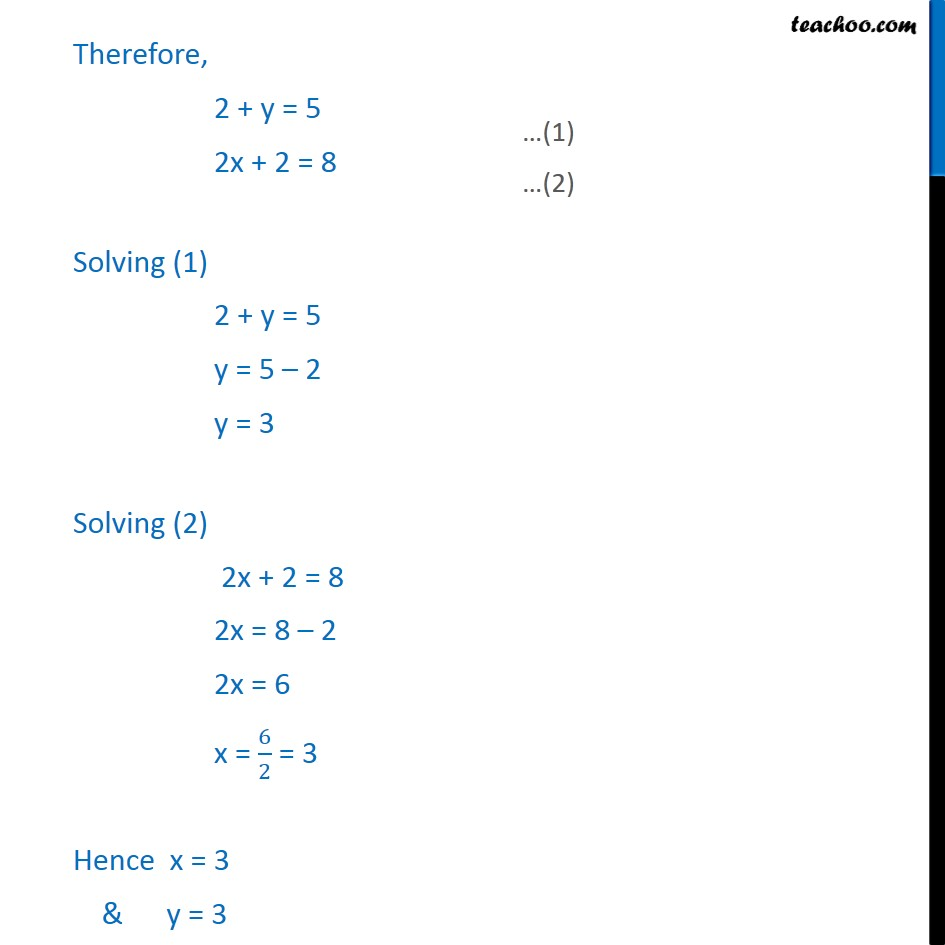1. Chapter 3 Class 12 Matrices (Term 1)
2. Serial order wise
3. Ex 3.2

Transcript

Ex 3.2,9 Find x and y, if 2[ 8(1&3@0& )] + [ 8( &0@1&2)] = [ 8(5&6@1&8)] Given that 2[ 8(1&3@0& )] + [ 8( &0@1&2)] = [ 8(5&6@1&8)] [ 8(1 2&3 2@0 2& 2)] + [ 8( &0@1&2)] = [ 8(5&6@1&8)] [ 8(2&6@0&2 )] + [ 8( &0@1&2)] = [ 8(5&6@1&8)] [ 8(2+ &6+0@0+1&2 +2)] = [ 8(5&6@1&8)] [ 8(2+ &6@1&2 +2)] = [ 8(5&6@1&8)] Since matrices are equal. Corresponding elements are equal Therefore, 2 + y = 5 2x + 2 = 8 Solving (1) 2 + y = 5 y = 5 2 y = 3 Solving (2) 2x + 2 = 8 2x = 8 2 2x = 6 x = 6/2 = 3 Hence x = 3 & y = 3

Ex 3.2